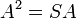# Approximate subgroup

Suppose$d$ is a positive integer. A finite subset$A$ of a group$G$ is termed a$d$-approximate subgroup if it is a symmetric subset containing the identity element such that there exists a subset$S$ of$G$ of size at most$d$ such that the product of subsets$A^2$ coincides with the product of subsets$SA$:$A^2 = SA$
where$A^2 = \{ a_1a_2 \mid a_1,a_2 \in A \}$ and$SA = \{ sa \mid s \in S, a \in A \}$
Note that any finite symmetric subset$A$ containing the identity element is always a$|A|$-approximate subgroup, and$A$ is a 1-approximate subgroup if and only if it is a subgroup. The minimum value of$d$ for which$A$ is a$d$-approximate subgroup describes how close$A$ is to being a subgroup.